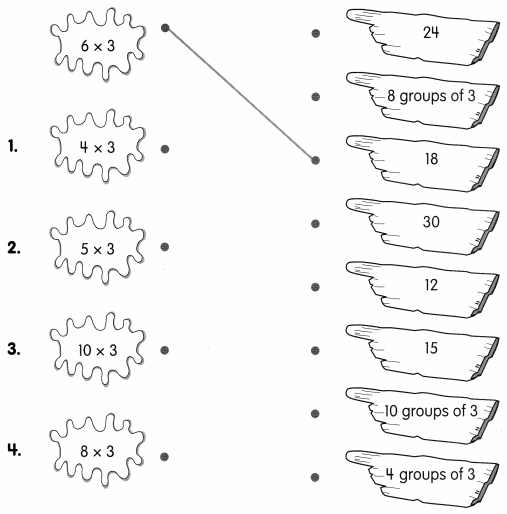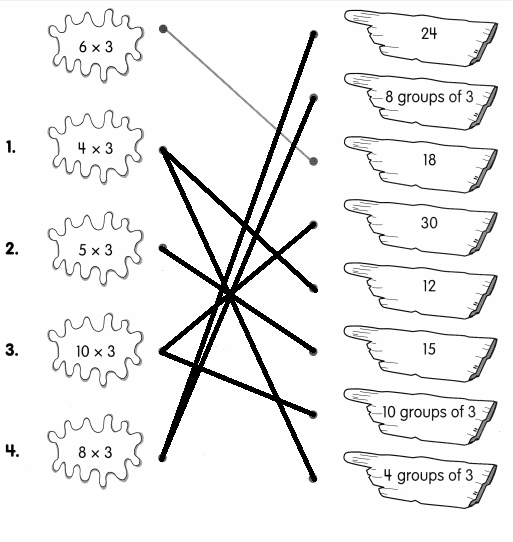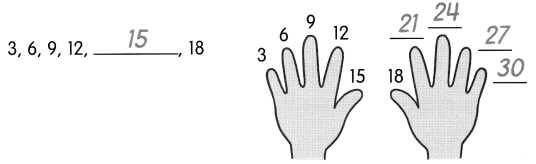# Math in Focus Grade 2 Chapter 15 Practice 1 Answer Key Multiplying 3: Skip-Counting

This handy Math in Focus Grade 2 Workbook Answer Key Chapter 15 Practice 1 Multiplying 3: Skip-Counting detailed solutions for the textbook questions.

## Math in Focus Grade 2 Chapter 15 Practice 1 Answer Key Multiplying 3: Skip-Counting

Match the shapes that have the same value.Count by 3s. Then fill in the blanks.

ExampleQuestion 5.
9, 12, 15, _________, _________, _________, _________

Question 6.
12, _________, 18, 21, _________, _________, 30

Fill in the blanks.

Question 7.
4 × 3 = _________
Answer: 4 x 3 = 12

Question 8.
2 × 3 = _________
Answer: 2 x 3 = 6

Question 9.
6 × 3 = _________
Answer: 6 x 3 = 18

Question 10.
8 × 3 = _________
Answer: 8 x 3 = 24

Question 11.
9 × 3 = _________
Answer: 9 x 3 = 27

Question 12.
7 × 3 = _________
Answer: 7 x 3 = 21

Question 13.
3 × 3 = _________
Answer: 3 x 3 = 9

Question 14.
10 × 3 = _________
Answer: 10 x 3 = 30

Solve.

Question 15.
Andrea has 5 flower vases. Each vase has 3 roses. How many roses are there in all?
5 × 3 = __________
There are __________ roses in all.
Answer: 5 x 3 = 15
There are 15 roses in all.